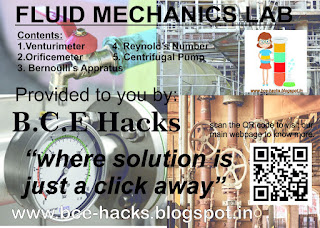USCT

## Fluid Mechanics LabFluid Mechanics Lab Third Semester

The Lab of Fluid Mechanics will provide you with the practical application of the fundamentals of Fluid Mechanics by the use of types of equipment like Venturimeter, Orifice Meter, Bernoulli's apparatus etc.

### Following are the experiments in Fluid Mechanics:

1. To Determine discharge coefficient of given Venturimeter and obtain its variation with Reynold's number.
2. To determine discharge coefficient of orifice meter and obtain its variation with Reynold's number.
3. To verify Bernoulli's theorem using Bernoulli's apparatus.
4. To study different types of flow regimes using Reynold's apparatus.
5.To study the characteristics of Centrifugal Pump.

### FREQUENTLY ASKED VIVA QUESTIONS OF FLUID MECHANICS:

#### Venturimeter:

Q1.What is venturimeter used for?
Answer. They can be applied to the measurement of gasses but they are mostly used for liquids.
The following is only limited to incompressible fluids.

Q2.What is the basic equation of venturimeter meter?
Answer. Venturimeter follows the Bernoulli's equation i.e.  P1/ρ + v12/2g  = P2/ρ + v22/2g

Q3.Why is the angle of downstream small in venturimeter?
Answer. To make the pressure recovery large, by which Boundary Layer separation is prevented and friction is minimised.

Q4.Define discharge coefficient.
Answer. It is the ratio of actual discharge to the theoretical discharge.
It is dimensionless.

Q5.Can Cd be used for both discharge coefficient of Venturi meter and Orifice meter?

Q6.Why is actual discharge less than theoretical discharge in Venturi meter?
Answer. if it is not viscous then actually is closer to theoretical but, due to discharge depends on velocity and losses also effect in form of frictional losses in pipes and fittings.

Q7.Difference between the coefficient of discharge and Venturi coefficient.
Answer.  The coefficient of discharge is coefficient of the valve. It depends on how the valve controls the discharge.

Q8. Draw the diagram of venturimeter.

#### Orifice Meter:

Q1. Describe the structure of Orifice meter.
Answer. The Orifice meter consists of a flat Orifice plate with a circular hole drilled in it. There is a pressure tap upstream from the Orifice plate and just another downstream.

Q2.  What is the basic equation for Orifice meter?
Answer. Orifice meter follows the Bernoulli's equation i.e.  P1/ρ + v12/2g  = P2/ρ + v22/2g

Q3. What are the basic differences between venturimeter and Orifice meter?
a) The Orifice meter can be easily changed to accommodate different flow rates, whereas throat diameter for a venturi meter is fixed.
b) The Orifice meter has a large permanent loss of pressure which venturimeter doesn't have due to its shape which reduces the formation of Eddies and greatly reduces the permanent loss.
c)  The Orifice meter is cheap and easy to install whereas Venturi meter is expensive, as it must be carefully proportioned and fabricated.
d) Venturi meter is only for permanent installations whereas the throat diameter of the orifice can be changed accordingly.

Q.4 Why is Orifice meter widely preferred over venturi meter?
Answer. Because of there greater flexibility, i.e. Installing a new Orifice plate with a different opening is a simpler matter.

Q.5 What is Vena contracta?
Answer. Vena contracta is the point in a fluid stream where the diameter of the stream is the least and fluid velocity is at its maximum, such as in the case of the stream issuing out of a nozzle.

#### Bernoulli's Apparatus:

Q1. What is Bernoulli's equation?
Answer. Bernoulli's equation can be given as P/ρ + v2/2g + z = constant or we can say that sum of pressure head, velocity head and datum head is constant.

Q2. What is the correction factor in Bernoulli's equation?
Answer. In case of real fluids, due to energy losses in overcoming frictional resistance, the Bernoulli's theorem for real fluids can be given as :
P1/ρ + v12/2g + z1 = P2/ρ + v22/2g + z2 + H1,2

Where H1,2  is heat loss from 1 to 2.

Q3. What are the assumptions of Bernoulli's equation?
Answer. Steady flow, Incompressible flow, Frictionless flow, No shaft work, No transfer of heat and valid for flow along a single streamline.

Q4. What is an ideal fluid?
Answer. Ideal fluid is a hypothetical fluid which is assumed to be non-viscous, non-rotational, and incompressible.

Q5. Give some examples of Newtonian and Non-Newtonian fluids.
Answer.       Newtonian fluid - air, water, kerosene
Non-Newtonian fluid - toothpaste, blood, slurry's

Q.6 Applications of Bernoulli's equation.
Answer. Bernoulli's equation can be used for  Sizing of pumps, Flow sensor, Ejectors, Carburetor and Pitot tube.

#### Reynolds Number:

Q1. Define Reynolds number.
Answer. Reynolds number is the dimensionless number that is inertial force per viscous force.
Re= ρVD / µ
It helps determine whether the flow is laminate or turbulent.

Q2.What will be the value of Reynolds number for an ideal fluid?

Since viscosity is zero for an ideal fluid, therefore Reynolds number will be infinity.

Q3. define the laminar and turbulent flow.
Laminar flow :
a.Fluid layer moves parallel to each other and does not cross each other
b. Fluid is flowing at low velocity.
c. The fluid flow is Laminar when the value of Reynolds number, Re is less than 2000.

Turbulent Flow:
a. It occurs when fluid flows in high-velocity
b. The fluid flow is Laminar when the value of Reynolds number, Re is greater than 4000

Q.4 What is transition state?
Answer. It is the stage between Laminar and turbulent flow.  i.e. 2100 < Re < 4000

#### Centrifugal Pump :

Q1. Define Pump.
Answer. The hydraulic machine which converts mechanical energy into hydraulic energy is known as the pump.

Q2. What is a Centrifugal Pump?
Answer. The machine that converts mechanical energy into fluid energy by centrifugal force on the fluid is called centrifugal pump.

Q3. Draw the diagram of Centrifugal Pump.

#fluid_mechanics #USCT #GGSIPU #practical #lab #third #semester